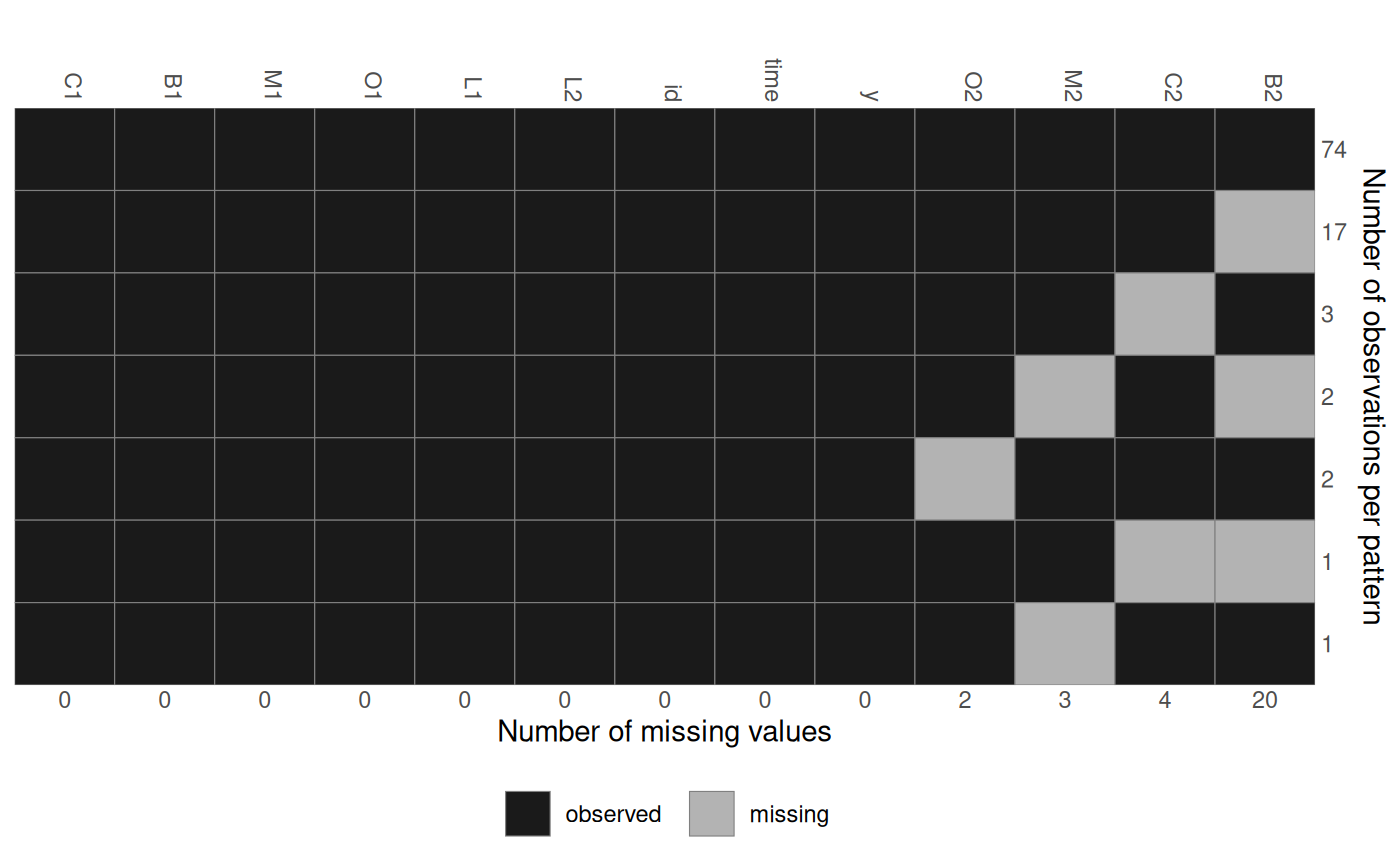Obtain a plot of the pattern of missing data and/or return the pattern as a matrix.

md_pattern(data, color = c(grDevices::grey(0.1), grDevices::grey(0.7)),
border = grDevices::grey(0.5), plot = TRUE, pattern = FALSE,
print_xaxis = TRUE, ylab = "Number of observations per pattern",
print_yaxis = TRUE, legend.position = "bottom", sort_columns = TRUE,
...)

## Arguments

data

data frame

color

vector of length two, that specifies the colour used to indicate observed and missing values (in that order)

border

colour of the grid

plot

logical; should the missing data pattern be plotted? (default is TRUE)

pattern

logical; should the missing data pattern be returned as matrix? (default is FALSE)

print_xaxis, print_yaxis

logical; should the x-axis (below the plot) and y-axis (on the right) be printed?

ylab

y-axis label

legend.position

the position of legends ("none", "left", "right", "bottom", "top", or two-element numeric vector)

sort_columns

logical; should the columns be sorted by number of missing values? (default is TRUE)

...

optional additional parameters, currently not used

## Note

This function requires the ggplot2 package to be installed.

op <- par(mar = c(3, 1, 1.5, 1.5), mgp = c(2, 0.6, 0))